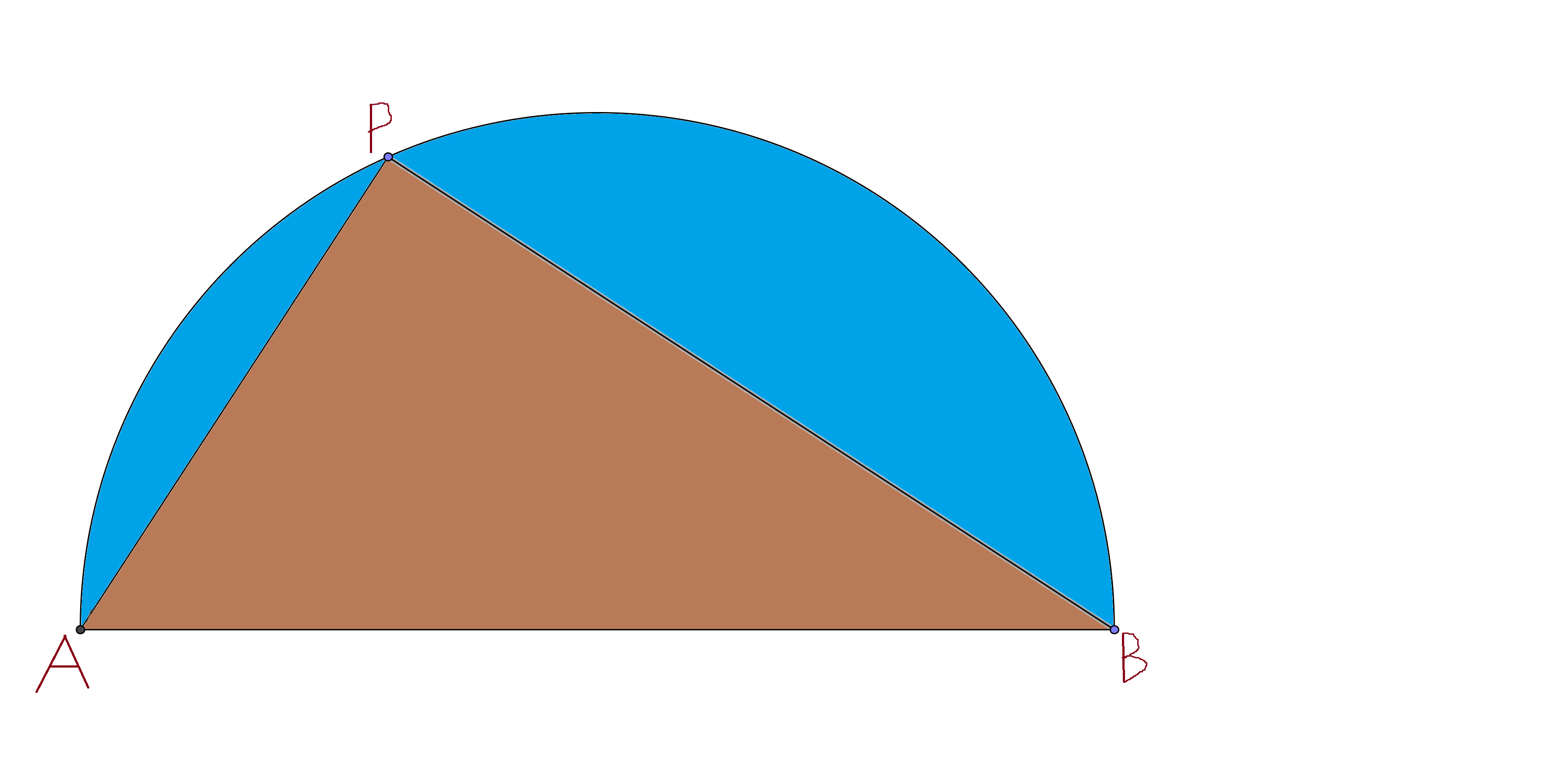# Seek Earth's Geography in Alg-Geometry

Geometry Level 3Consider a semicircle with radius $r$ and a point $P$ on its circumference such that $PA + PB$ is maximum.

Then, find the area of the blue region if $r=6370$. (Radius of earth is 6370 km)

Use the approximation $\pi = \dfrac{22}{7}$.

×

Problem Loading...

Note Loading...

Set Loading...